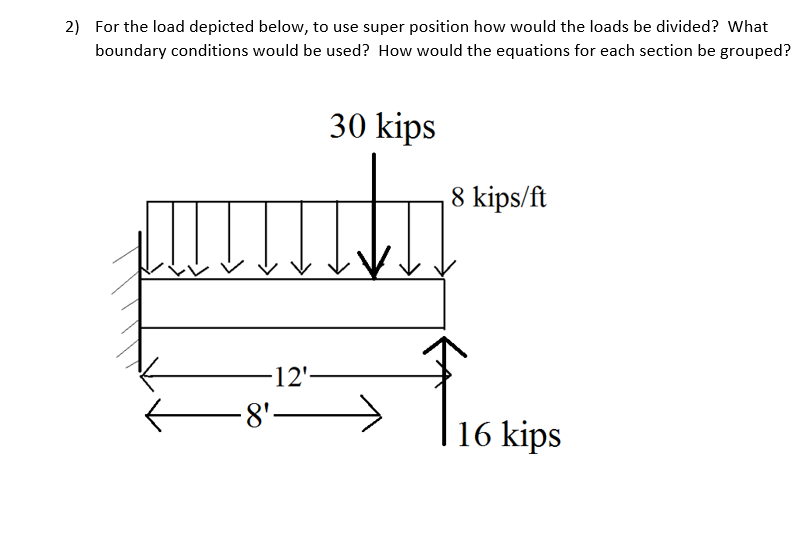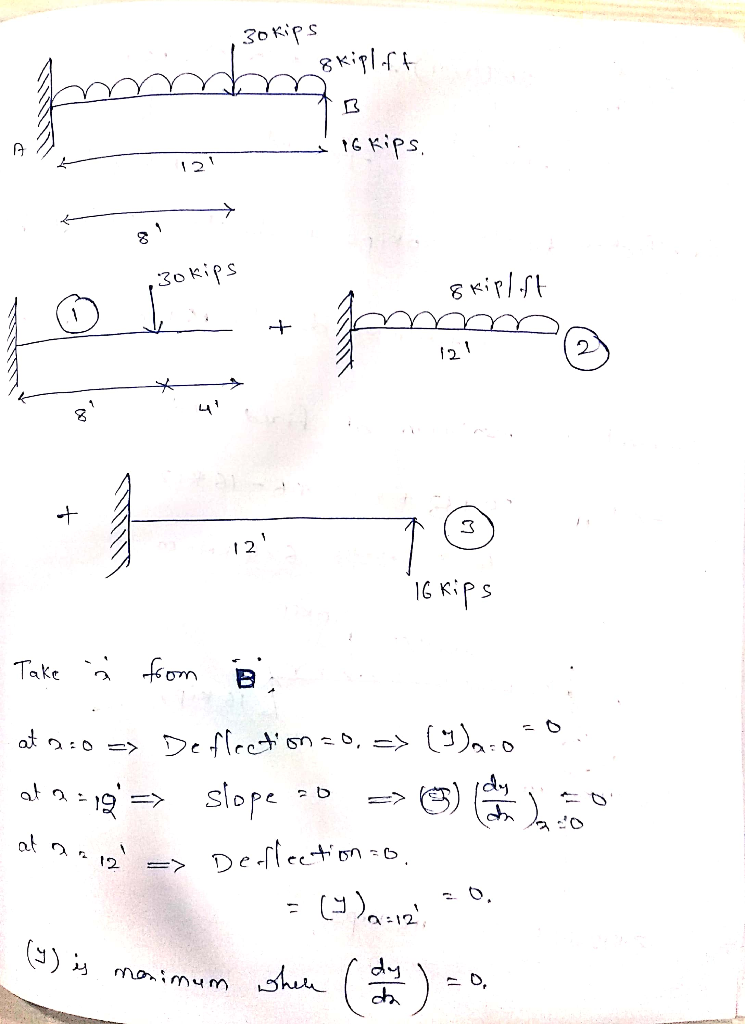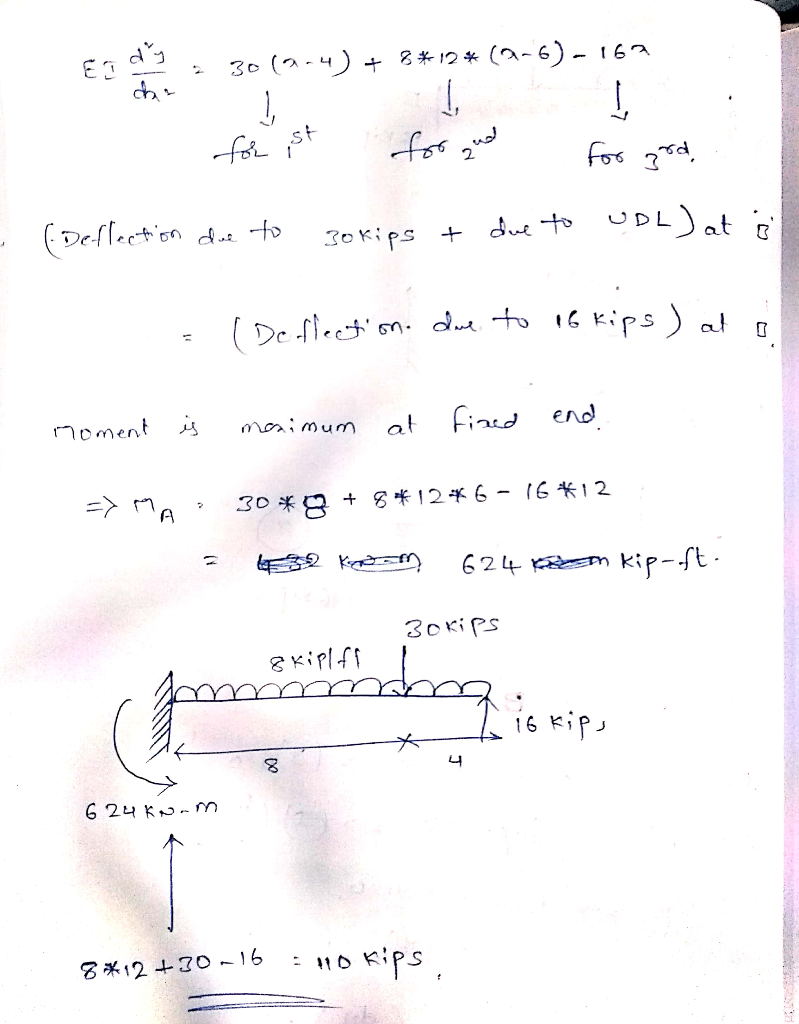Homework Help Question & Answers

For the load depicted below, to use super position how would the loads be divided? What boundary ...For the load depicted below, to use super position how would the loads be divided? What boundary conditions would be used? How would the equations for each section be grouped? 2) 30 kips 18 kips/ft 12 8'--> 1 16 kipsAdd Answer of: For the load depicted below, to use super position how would the loads be divided? What boundary ...
More Homework Help Questions Additional questions in this topic.

• Design a square tied column to carry axial service loads of 320 kips dead load and 190 kips live load. There is no identified applied moment. Assume that the column is short. Use f’c =4000 psi and fy...

Need Online Homework Help?

Get FREE EXPERT Answers
WITHIN MINUTES
Related Questions